››› Screenshot

THE SLIDE RULE OF SILICON DESIGN

Free Analog Circuit Simulation

Noise Analysis (Simple Example)

Operating point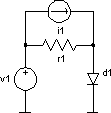noise example .control noise v(2) v1 lin 8 100kHz 1MegHz 2 .endc v1 1 0 dc 700mV ac 1V i1 1 2 dc 10mA ac 50mA r1 1 2 1 d1 2 0 diode .model diode d rs=10m tt=5us kf=1f .end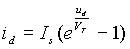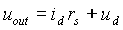Linearised ac circuit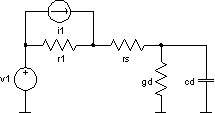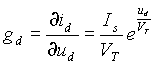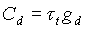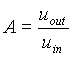Note, that ac magnitude of the input source has to be one. Thermal noise of the resistors r1 and rs given by: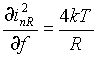Shot and flicker noise of the diode d1 given by: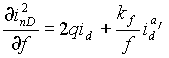All independent sources eliminated (set to zero), noise generators added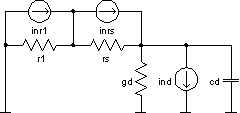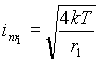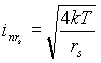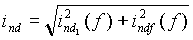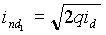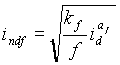Noise analysis creates two plots named noise1 and noise2. The first one contains the information about noise spectral densities, and the second about total noises. The vectors in noise1 are:

onoise_r1=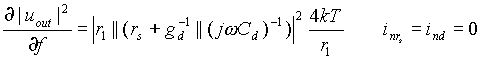onoise_d1_rs =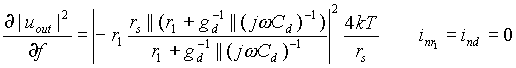onoise_d1_id =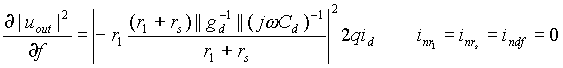onoise_d1_1overf =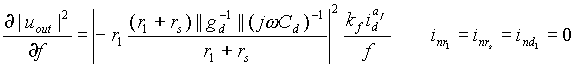onoise_d1 = onoise_d1_rs + onoise_d1_id + onoise_d1_1overf
onoise_spectrum = onoise_r1 + onoise_d1
inoise_spectrum = onoise_spectrum / |A|2

The vectors in noise2 are:

 onoise_total_r1 =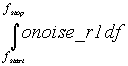onoise_total_d1_rs =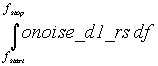onoise_total_d1_id =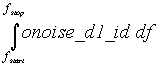onoise_total_d1_1overf =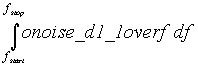onoise_total_d1 =onoise_total_d1_rs + onoise_total_d1_id + onoise_total_d1_1overf
onoise_total = onoise_total_r1 + onoise_total_d1

 inoise_total_r1 =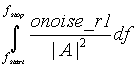inoise_total_d1_rs =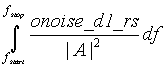inoise_total_d1_id =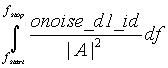inoise_total_d1_1overf =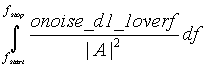inoise_total_d1 =inoise_total_d1_rs + inoise_total_d1_id + inoise_total_d1_1overf
inoise_total = inoise_total_r1 + inoise_total_d1